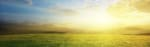All About Creation# Age Of The Earth

Age of the Earth: How Can We Know?
Can we determine the age of the earth, and if so, how? Scientists generally agree that the answer to the riddle of the age of the earth is carefully concealed within the earth's crust. Thus, the geologic timescale and radiometric dating have been developed in an effort to determine the age of the earth. The older of the two dating methods, the geologic timescale, is actually a circular argument and is therefore considered by many scholars to be weak. Nevertheless, the geologic timescale was thought to have been redeemed and refined with the advent of radiometric dating. Radiometric dating is more objective, and thus, more substantial. However, there are some underlying assumptions to consider.

Age of the Earth - The Geologic Column
The geologic column is the older of the two dating methods employed by scientists to determine the age of the earth. Basically, this is how it works: earth's many rock layers contain billions of fossils. Certain fossils are unique to certain layers of rock. Some of these fossils have been chosen to be what are called "index fossils". Scientists assume the age of an index fossil by the stage of evolutionary history the fossil is assumed to be in. That age is then transferred to the rock layer in which the index fossil was found. Then, to determine the age of all the other fossils in the same rock layer, we look at the age of the rock layer in which they are contained. Thus, we determine the age of the rock by the fossils it contains, and we determine the age of the fossils by the rock in which they are found. Many consider this circular reasoning. To learn more about this circular argument, explore our geologic time scale site.

Age of the Earth - Radiometric Dating
Radiometric dating is the primary dating scheme employed by scientists to determine the age of the earth. In a nutshell, this is how it works: atoms are generally regarded as the smallest unit of matter; everything is made of atoms. Atoms consist of protons, neutrons and electrons. An "element" is a substance made up of atoms which have the same number of protons. However, if these atoms have too many or too few neutrons, the element is unstable and will decay. The unstable element will eventually decay into a stable element. Radiometric dating techniques utilize this natural decay process by measuring how long it takes for the unstable element to decay into a stable element and by measuring how much stable element has been produced by the unstable element, thus determining how long the unstable element has been decaying. An age is then transferred to the specimen of rock containing the measured elements. The popular age of 4.6 billion years old for the planet earth was derived by applying radiometric dating techniques to a meteorite called "allende" (a-yen-day), which scientists assume formed at the same time as the earth. However, this date is not conclusive. Certainty and assumption are contrary. The dates derived by radiometric dating techniques are highly publicized but the fundamental assumptions employed are not. To learn more about these foundational assumptions, explore our radiometric dating site.

Investigate More Now!